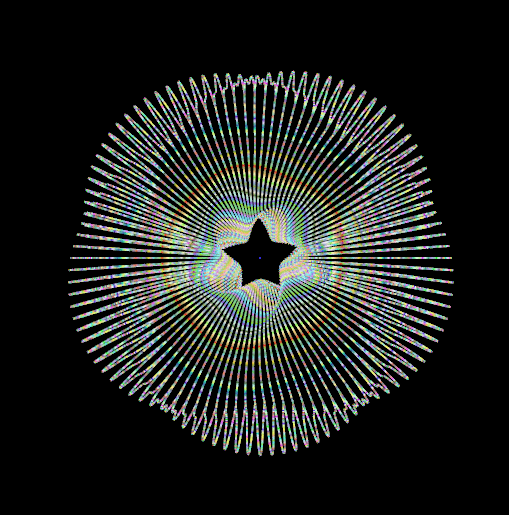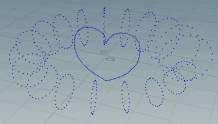# Infinite knot

## Recommended Posts

@mike_Just rotate .. with this you have endless possibility ...Have Fun ..use after add SOP or skin or copy to points that Star Shape.

```//detail
int u = chi('u_num');
int v = chi('v_num');
float inner = chf('inner');
float outer = chf('outer');
float pi = \$PI *2;

for(int i=0; i<u; i++){
for(int j=0;j<v;j++){
float centerX = inner +(outer -inner)*0.5;
float X = centerX +torusRad *cos(pi / u *i);
float Y = torusRad *sin(pi / u *i);
vector pos = set(X*cos(pi / v*j),Y,X *sin(pi /v *j));

setpointattrib(0,'u',pt,i);
setpointattrib(0,'v',pt,j);

vector centerPt = set(centerX *cos(pi / v *j), 0,centerX *sin(pi /v *j));
vector normal = normalize(pos -centerPt);
setpointattrib(0,'N',pt,normal);
}
}```
```int ucount = chi('ucount');
int vcount = chi('vcouont');
float height = chf('height');
float waveslid = chf('waveslid');

int uWaveCount = chi('uWaveCount');
int vWaveCount = chi('vWaveCount');

int u = point(0,'u',@ptnum);
int v = point (0,'v',@ptnum);

vector nor =v@N;

float uRatio = u /float(ucount);
float vRatio = v /float(vcount);
float pi = \$PI * 2;

vector newPos = @P + nor *height * sin(pi * vRatio *vWaveCount + pi * uRatio *uWaveCount + pi * waveslid);
@P = newPos;```

sec wrangleEdited by Librarian
•1
•1
##### Share on other sites

@Librarian Oh Wow , thanks a lot !

•1
##### Share on other sites

I installed the two wrangle, and I'm getting some action, but it doesn't really look like my supplied heart shape. Can you recommend any settings for the two wrangles?ap_lib_infinite_knot.hiplc

## Join the conversation

You can post now and register later. If you have an account, sign in now to post with your account.
Note: Your post will require moderator approval before it will be visible.×   Pasted as rich text.   Paste as plain text instead

Only 75 emoji are allowed.

×   Your previous content has been restored.   Clear editor

×   You cannot paste images directly. Upload or insert images from URL.

×
×